4.5 TWO-FIRM ANALYSIS

Suppose you invest in two securities only. This simplifies the general problem because it turns out that every portfolio lies on the boundary of the scatter plot in Figure 4.2. (This changes as soon as you have three or more securities.) The two-security case lets you see how varying the portfolio weights alters the risk/return characteristics of a portfolio. This is an important building block toward the goal of understanding the general problem.

Formally, the expected return from investing in Firms 1 and 3 in the Three-Firm Case may be expressed as.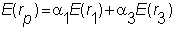Because portfolio shares must add up to one, a1 + a3 must equal one. That is, if Firm 1 has a weight of 0.3, then Firm 3 has a weight of (1 - 0.3) = 0.7. The sum of their respective expected returns multiplied by their weights gives the portfolio return. Because the portfolio weights sum to one, we can eliminate the weight on Firm 3 and write the expected portfolio return as a linear function of a single portfolio weight: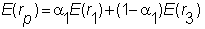This equation can then be rearranged into the general form of a straight line.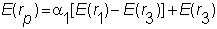In this form, the slope of the expected return line is the difference between the expected return on Firms 1 and 3, and the intercept is the expected return on Firm 3.

This form is useful to show you why the problem of computing the frontier is quite simple in the case of two Firms. Recall that the minimum-variance frontier tells you the smallest variance for a target expected return. If E is the target expected return, then the portfolio that yields this target must have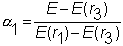In fact, this is the only portfolio that gives the target return. Therefore its variance is automatically the minimum-variance for this target return. This makes the problem of computing the minimum-variance frontier quite simple.

Of course, we can solve for the weight of Firm 1 in this way because there are only two securities. With three or more securities, it is a more complicated problem.

We turn next to the effect of changing portfolio weights on risk. The risk for a two-firm portfolio is measured by the portfolio's standard deviation. This equals: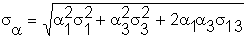In the right-hand side of this expression, the first two terms are weighted variance terms, and the last term is a weighted covariance term. Equivalently, as developed in Chapter 2, on population statistics or sample statistics, the covariance term divided by the product of each firm's standard deviation, is a number scaled between -1, and 1. This number is called the correlation coefficient and it denotes as r for the sample coefficient or r for the population coefficient.

If we substitute the sample correlation coefficient into the portfolio risk equation, the relationship between portfolio risk and correlation becomes quite transparent.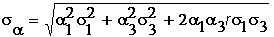Two critical variables that affect the attainable points in risk/return space are :

1. The correlation coefficient between Firms 1 and 3.

2. The value of a1. If a1 is allowed to become negative or greater than 1, then short selling is permitted.

What we want to do is construct the minimum-variance frontier. We start by disallowing short selling, and then by permitting short selling. Recall that the basic parameters for Firms 1 and 3 in the Three-Firm Case are :

Firm 1: E(Return) = 0.229, Risk (standard deviation) = 0.961.

Firm 3: E(Return) = 0.052, Risk (standard deviation) = 0.727.

Correlation coefficient = -0.833.

No Short Selling

The correlation is negative, so you can see that we get to subtract away some number from the first two terms in the square root formula below. This immediately shows you the power of negative covariance or correlation for reducing portfolio risk: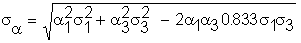Using the default data set you may want to experiment with CAPM Tutor to see how small you can make this standard deviation by varying only the weights of Firms 1 and 3. On doing this, you will observe that you are unable to eliminate all portfolio risk.

This occurs because the correlation is strictly in between -1 and 1. If r = -1, then it is possible to eliminate all risk when short selling is not allowed. To see this, suppose the correlation actually equals -1.

Replacing -0.833 with -1 yields: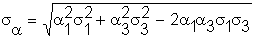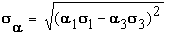This can be made zero by choosing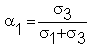.

You can work out that if the correlation coefficient is zero (i.e., the two returns are independent of each other), then it is impossible for the portfolio risk to be eliminated.

Finally, if the correlation coefficient equals +1, and short selling is not permitted, you can work out that it is again impossible to eliminate all risk.

Short Selling

If short selling is permitted, then once again all risk can be eliminated if the correlation is either -1 or +1. If the correlation is -1, then the portfolio without short sales will do. If it is +1, then the standard deviation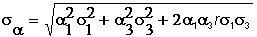,

reduces to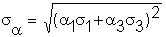and thus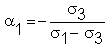will eliminate all risk.

The next part of this chapter lets you read about the general case in the topic N-Security Case.

previous topic

next topic